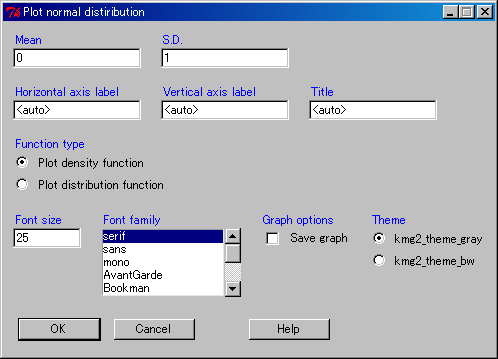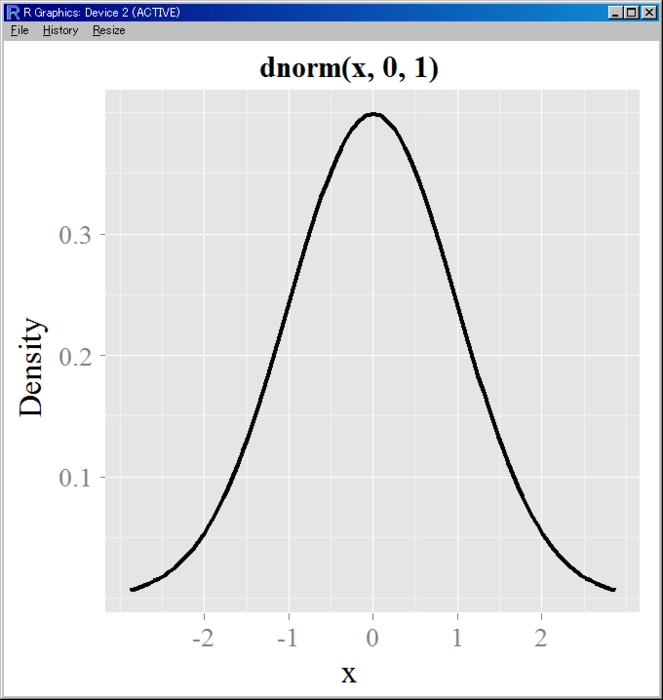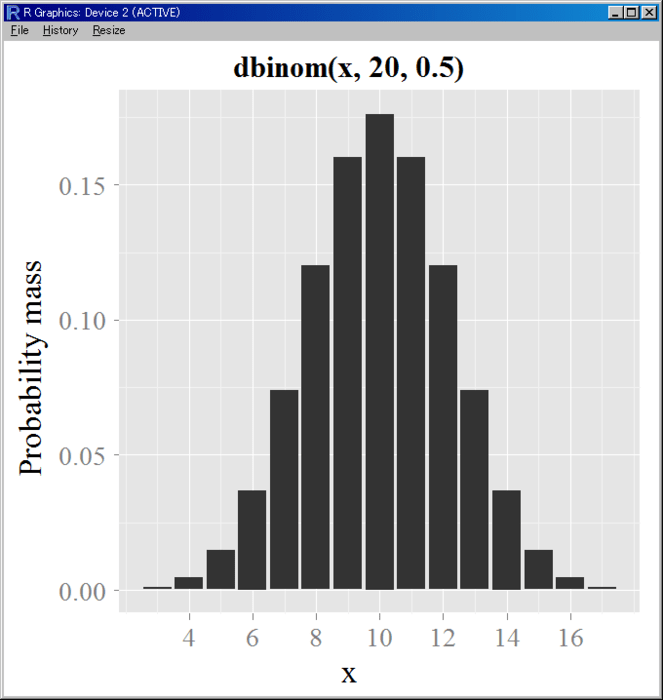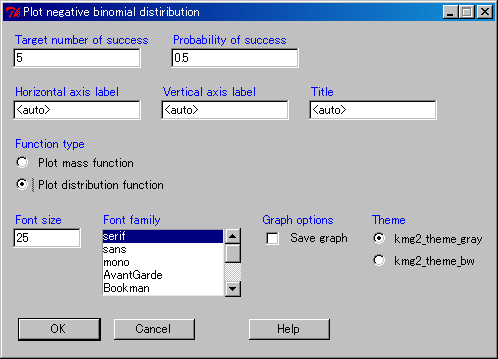Want to share your content on R-bloggers? click here if you have a blog, or here if you don't.

RcmdrPlugin.KMggplot2 (CRAN)

I posted an Rcmdr plug-in for a “ggplot2” GUI front-end on CRAN.

This version supports Kaplan-Meier plot and other plots as follow:

• Kaplan-Meier plot
• Show no. at risk on inside
• Show no. at risk table on outside
• Histogram
• Color coding
• Density estimation
• Q-Q plot
• Create plots based on a maximum likelihood estimate for the parameters of the selected theoretical distribution
• Create plots based on a user-specified theoretical distribution
• Box plot / Errorbar plot
• Box plot
• Mean ± S.D.
• Mean ± S.D. (Bar plot)
• 95% Confidence interval (t distribution)
• 95% Confidence interval (bootstrap)
• Scatter plot
• Fitting a linear regression
• Smoothing with LOESS for small datasets or GAM with a cubic regression basis for large data
• Scatter plot matrix
• Fitting a linear regression
• Smoothing with LOESS for small datasets or GAM with a cubic regression basis for large data
• Line chart
• Normal line chart
• Line char with a step function
• Area plot
• Pie chart
• Bar chart for discrete variables
• Contour plot
• Color coding
• Heat map
• Distribution plot
• Normal distribution
• t distribution
• Chi-square distribution
• F distribution
• Exponential distribution
• Uniform distribution
• Beta distribution
• Cauchy distribution
• Logistic distribution
• Log-normal distribution
• Gamma distribution
• Weibull distribution
• Binomial distribution
• Poisson distribution
• Geometric distribution
• Hypergeometric distribution
• Negative binomial distribution#### Kaplan-Meier plot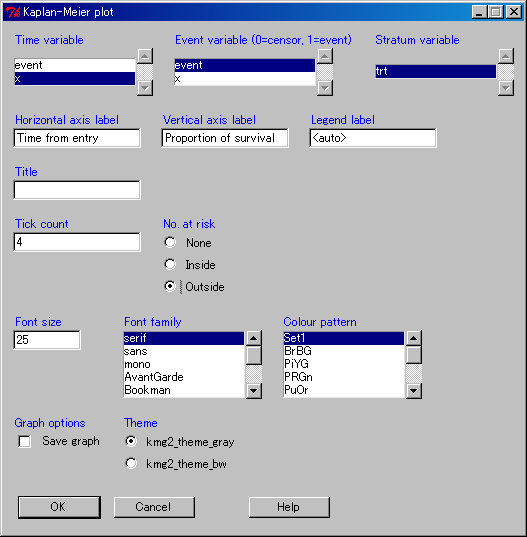#### Histogram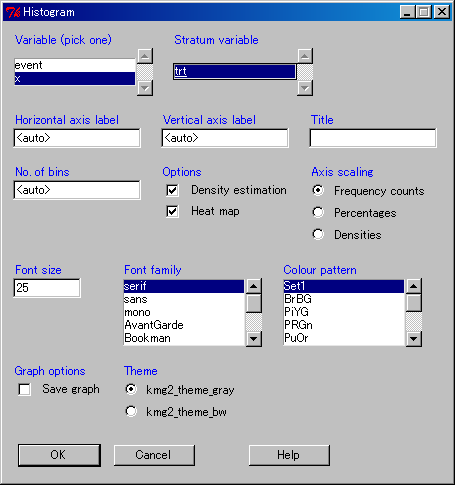#### Q-Q plot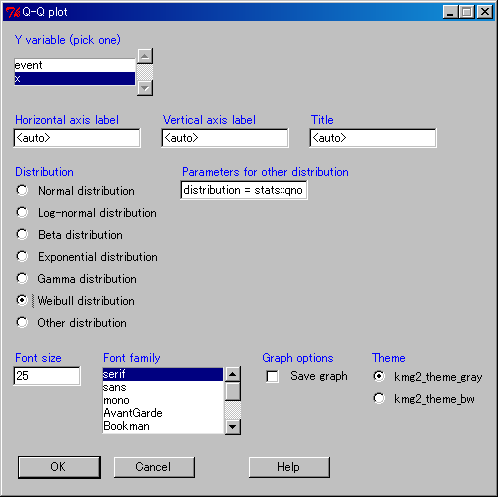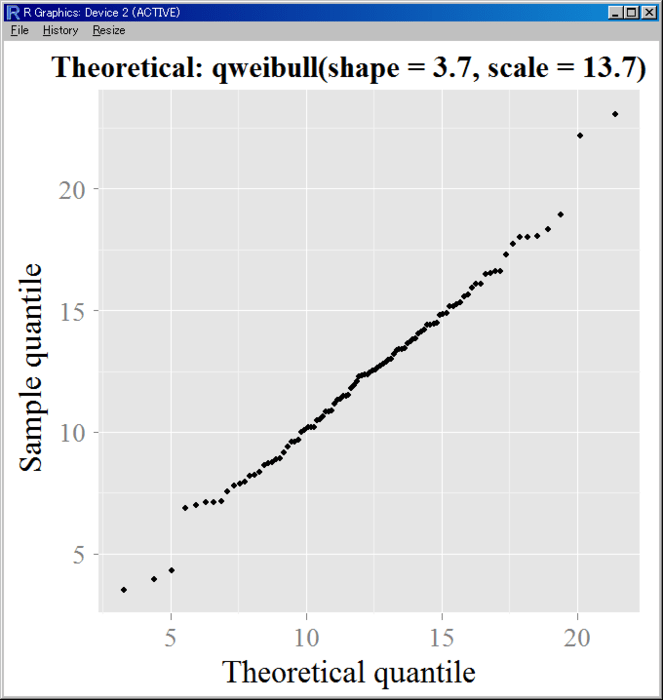#### Box plot / Errorbar plot#### Scatter plot#### Scatter plot matrix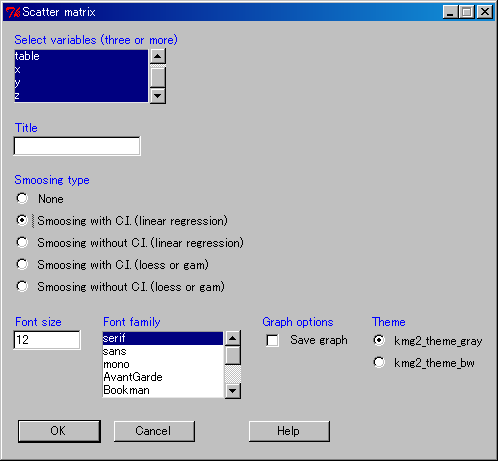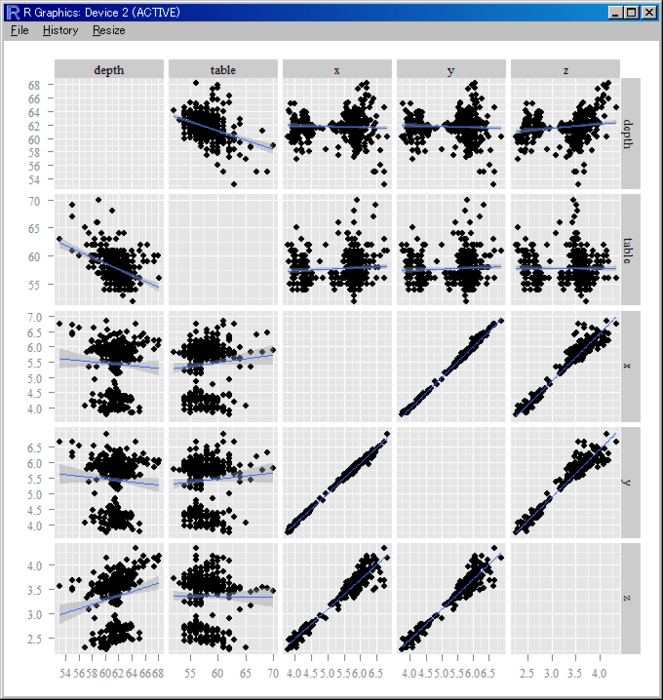#### Line chart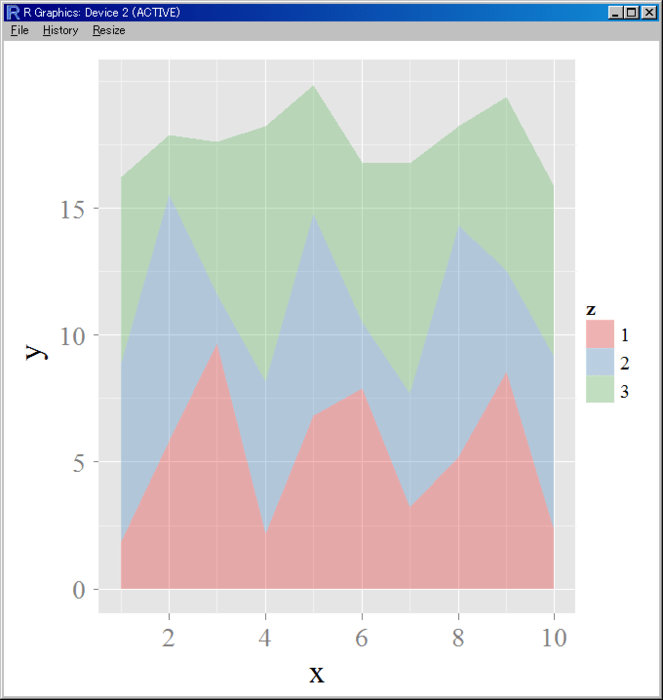#### Pie chart#### Bar chart for discrete variables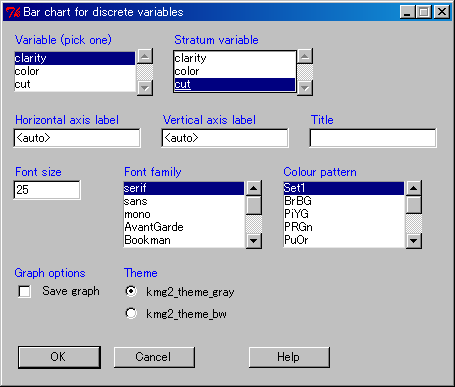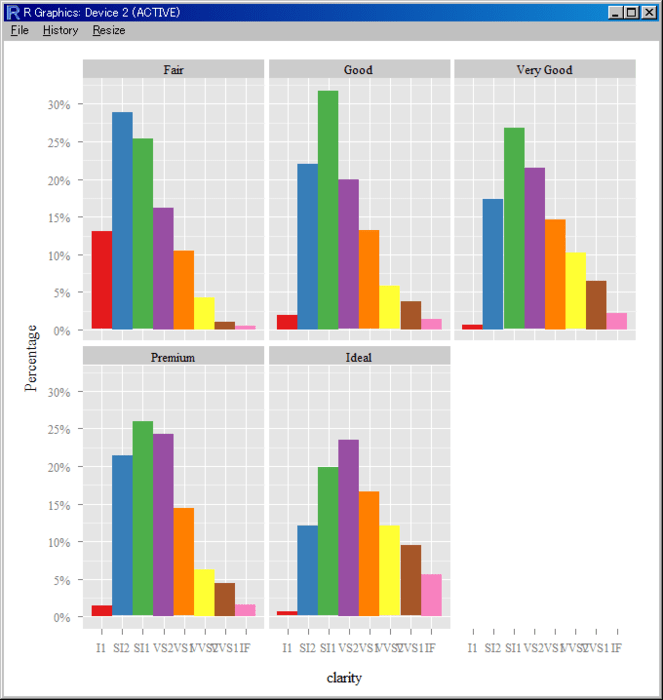#### Contour plot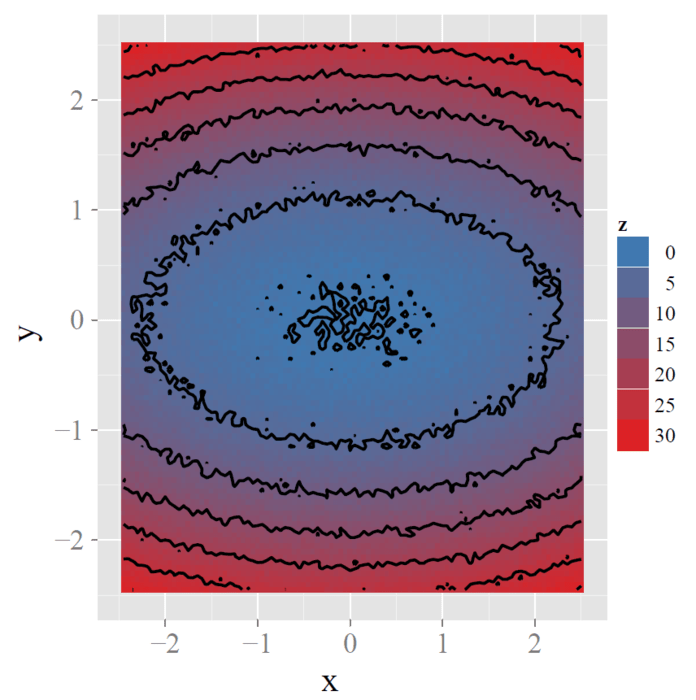#### Distribution plot